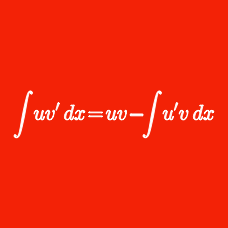Calculus

# Integration U-substitution - Problem Solving

When $$a=2$$ and $$b=4,$$ $$\int_{a}^{b}{\sqrt{(x-a)(b-x)}}dx$$ is equal to $$\underline{\qquad}.$$

For $$n$$ a positive integer, what is the indefinite integral $\int{ x{(1-x)}^{n+6} }dx?$

Details and assumptions

Use $$C$$ as the constant of integration.

Evaluate $\int_{0}^{\sqrt{3}} (x+4)^2 e^{x^2} dx+\int_{\sqrt{3}}^{0} (x-4)^2 e^{x^2} dx.$

Given that $$\displaystyle \int_0^4 x^3\sqrt{9+x^2} dx = a$$, what is the value of $$\lfloor a \rfloor$$?

Details and assumptions

Greatest Integer Function: $$\lfloor x \rfloor: \mathbb{R} \rightarrow \mathbb{Z}$$ refers to the greatest integer less than or equal to $$x$$. For example $$\lfloor 2.3 \rfloor = 2$$ and $$\lfloor -3.4 \rfloor = -4$$.

Determine the value of $$100 \int_0^{\pi} \sqrt{ 1 + \sin x} \, dx$$.

This problem is proposed by Cody.

×

Problem Loading...

Note Loading...

Set Loading...